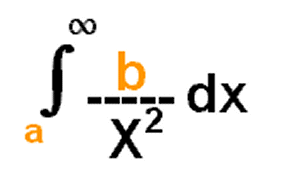ResourcesDepartment home page for MATH 260Textbook webpageA quick review to (Substitution , Integration by Parts, Partial Fractions, Trig Substitution)Integration FormulaThe Integrator uses webMathematica technology to bring the computational capabilities of Mathematica to the web. The heart of The Integrator is Mathematica's built-in Integrate function--the same one you use when accessing Mathematica directly. When you type in an integral, The Integrator uses webMathematica to send the integral to the Mathematica kernel, which computes the result using the Integrate function. The result is then embedded into the web page as typeset output.Step-by-Step Derivatives This example, provided by www.calc101.com, demonstrates how webMathematica creates educational calculators. This calculator evaluates derivatives step-by-step.